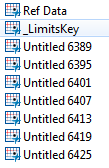Our World Statistics Day conversations have been a great reminder of how much statistics can inform our lives. Do you have an example of how statistics has made a difference in your life? Share your story with the Community!
Choose Language Hide Translation Bar
Highlighted

## Joining Multiple Data Table using a loop

Hi there,

Currently as part of my analysis I am creating multiple files based on control chart limits.These files are outputted as Untitled. I need to join all the analysis together but the problem that I have is that I need to exclude some data tables from my join. There are 2 tables that I need to exclude from my loop - Ref Data and Limits_Key.

See Below:I was using this code to place all the tables into a list:

num_tables = ntable();

dataset_list = {};

For (z = 1, z <= num_tables, z++,

dt = Data Table(z);

column_names = dt << get column names;

valid_columns = 1;

if (valid_columns,

one_name = dt << Get Name;

Insert Into(dataset_list, one_name);

);

);    // end for

//show(dataset_list);

num_tables = nitems(dataset_list);

For( q = num_tables, q >= 1, q--,

If( !Starts With( dataset_list, "Untitled" ),

Remove From( dataset_list, q )

)

);

Show(dataset_list);

I was hoping to use something like the following to join the tables (excluding the 2 mentioned above)

t_count = (N Table());

show (t_count);

t_count = (t_count) -2;

dt_old = Data Table(t_count);

show(dt_old);

Show(dt_old << get name());

dt_new = Data Table("temp_ref");

For(i = 3, i <= t_count, i++,

//show(Data Table);

dt_old = dt_new;

Show(dt_old << get name());

dt_new = dt_old << Join(

With(Data Table(i)),

Merge Same Name Columns,

By Matching Columns(:_LimitsKey = :_LimitsKey,

:_Screened = :_Screened,

:SUBSET = :SUBSET),

Drop multiples(0, 0),

Name("Include non-matches")(1, 1),

Output Table( "Limits" )

);

Close(dt_old);

);

Could anyone help me out or give me a few pointers?

1 ACCEPTED SOLUTION

Accepted Solutions
Highlighted

## Re: Joining Multiple Data Table using a loop

You can filter out unneeded tables in the very first loop.  You'll end up with a table called LimitsNNN, where NNN is the number of Untitled tables.  Try something like this.

num_tables = N Table();

dataset_list = {};

For( z = 1, z <= num_tables, z++,

dt = Data Table( z );

one_name = dt << Get Name;

If( Starts With( one_name, "Untitled" ),

Insert Into( dataset_list, one_name )

);

);    // end for

Show( dataset_list );

dt_old = data table(dataset_list);

for (i = 2, i <= nitems(dataset_list), i++,

dt_new = data table(dataset_list[i]);

join_name = "Limits" || char(i);

dt_old << join( with(dt_new),

Merge Same Name Columns,

By Matching Columns(

:_LimitsKey = :_LimitsKey,

:_Screened = :_Screened,

:SUBSET = :SUBSET

),

Drop multiples( 0, 0 ),

Name( "Include non-matches" )(1, 1),

Output Table( join_name )

);

close(dt_old, nosave);

dt_old = data table(join_name);

);

Highlighted

## Re: Joining Multiple Data Table using a loop

You can filter out unneeded tables in the very first loop.  You'll end up with a table called LimitsNNN, where NNN is the number of Untitled tables.  Try something like this.

num_tables = N Table();

dataset_list = {};

For( z = 1, z <= num_tables, z++,

dt = Data Table( z );

one_name = dt << Get Name;

If( Starts With( one_name, "Untitled" ),

Insert Into( dataset_list, one_name )

);

);    // end for

Show( dataset_list );

dt_old = data table(dataset_list);

for (i = 2, i <= nitems(dataset_list), i++,

dt_new = data table(dataset_list[i]);

join_name = "Limits" || char(i);

dt_old << join( with(dt_new),

Merge Same Name Columns,

By Matching Columns(

:_LimitsKey = :_LimitsKey,

:_Screened = :_Screened,

:SUBSET = :SUBSET

),

Drop multiples( 0, 0 ),

Name( "Include non-matches" )(1, 1),

Output Table( join_name )

);

close(dt_old, nosave);

dt_old = data table(join_name);

);

Article Labels

There are no labels assigned to this post.